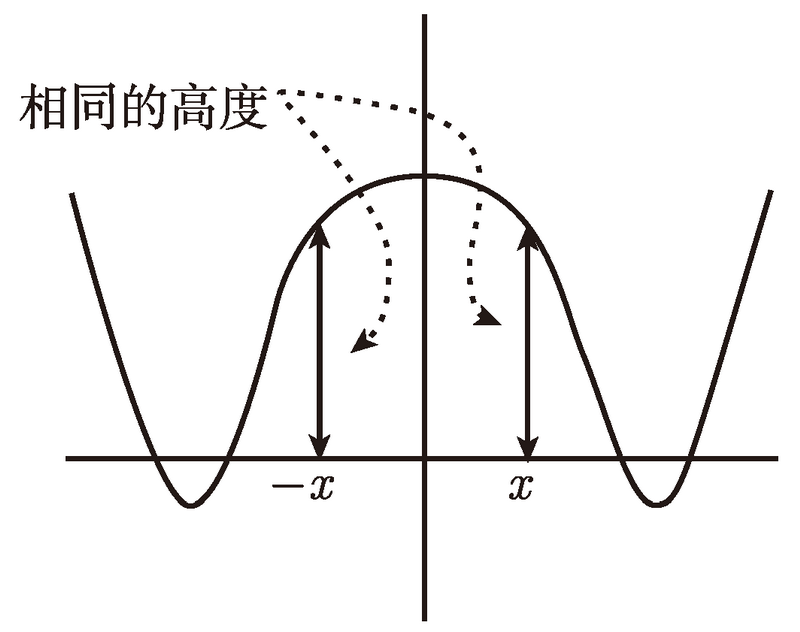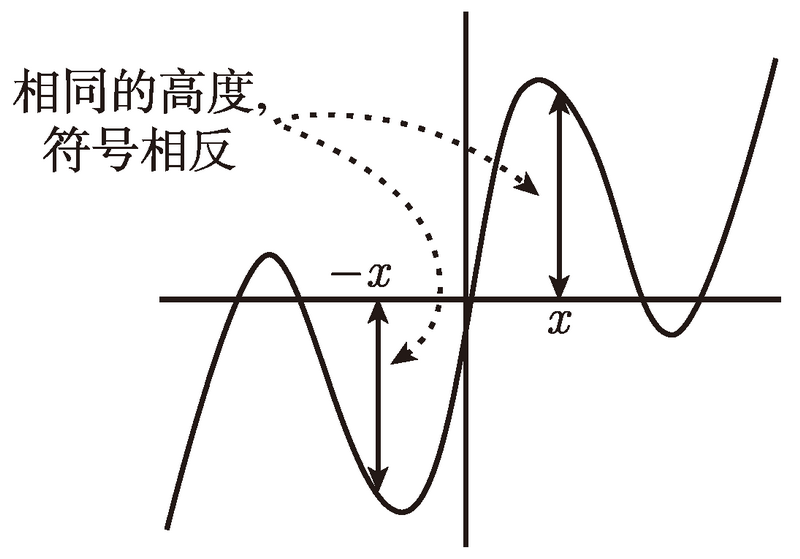# 普林斯顿微积分读本（修订版）(12)：函数、图像和直线 1.4(奇函数和偶函数)$f(-x)=\log_5(2(-x)^6-6(-x)^2+3)=\log_5(2x^6-6x^2+3),$

$g(x)=\frac{2x^3+x}{3x^2+5}$$h(x)=\frac{2x^3+x-1}{3x^2+5}$

$g(-x)=\frac{2(-x)^3+(-x)}{3(-x)^2+5}=\frac{-2x^3-x}{3x^2+5}.$

$g(-x)=\frac{2x^3+x}{3x^2+5},$

$h(-x)=\frac{2(-x)^3+(-x)-1}{3(-x)^2+5}=\frac{-2x^3-x-1}{3x^2+5}.$

$h(-x)=\frac{2x^3+x+1}{3x^2+5}.$

$h(-x)=f(-x)g(-x)=(-f(x))(-g(x))=f(x)g(x)=h(x).$